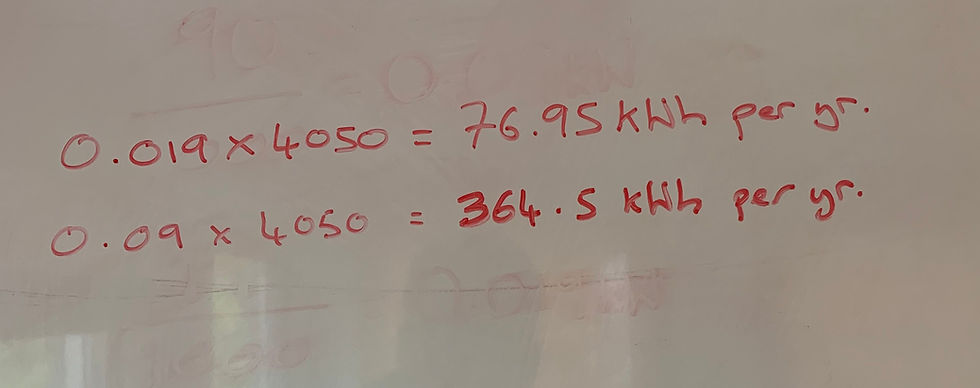top of page
Search
• Peter

# 'Energy Calculations - Street Lighting'

Reducing the energy consumption of the street lighting stock / asset is usually one of the big considerations when deciding on how to invest money in to the asset.

You see a trade off - capital investment for the return on revenue savings. Reducing the energy bill and the costs associated with maintaining the asset.

This has caused a big push to replace old lanterns with new LED street lights.

Energy Calculations:

To give an idea how you can estimate the potential savings with an LED replacement scheme we've jotted down below some simple equations you can use to estimate the energy reduction.

Street Light A represents the existing asset and Street Light B represents a possible replacement LED lantern:

Street Light A - 70W SON (90W circuit watts; includes gear etc)

Street Light B - 19W LED (19W circuit watts)

Simple starting point is to note that by replacing unit A we reduce the power consumption by 71W.

Power Usage:

Let's calculate the power usage in kilowatts:Street Light A: 0.09kW

Street Light B: 0.019kW

Yearly Energy Consumption:

Next we can work out the number of kWh each asset consumes per year.

To do this we need to know the number of hours the light will operate each year - if you operate a CMS system this can be reported and calculated for you. However, as a simple exercise we can refer to the Elexon burning hours for each particular region (sun set to sun rise).

In our example we will use a 4,050 hours per year. Calculation below:Light A = 364.5kWh per year

Light B = 76.95kWh per year

Reducing the yearly energy consumption by 287.55kWh

Energy Costs:

If we know the price per kWh, we can calculate the monetary reduction.

For this example we will work on the basis of 10p per kWh.

With this replacement we could potentially save £28.75 per year.

Carbon Usage:

If required we could also calculate the carbon usage of each of the above lightsWe hope this has assisted you in someway. If you have any queries regarding this, please get in touch.

All the best,

Peter

bottom of page